ONE SAMPLE T-TESTS

ONE SAMPLE T-TEST OF MEANS: Univariate Inference for Interval/Ratio Data

A. Large samples with a known population mean.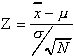Most of the time, the population's standard deviation is not available, so it is substituted by the sample's standard deviation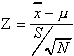.

where: N = total number in the sample.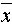= mean of the sample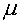= population mean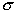= population standard deviation

S = sample standard deviation

Assumptions: N>100

Population mean known

Example: Anecdotal evidence indicates that students in 2:00 classes work less hours in the labor force than other students. The average student at the college works 23.8 hours a week. A sample of 147 students in 2:00 classes has a mean average of 22.47 hours a week and a standard deviation of 8.15 hours.

Q: Is the difference shown in the sample generalizeable to the whole population of students at the college?

A: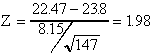Since there is a direction to the hypothesis, "less hours" indicates a negative relationship, the one-tail Z-score is used.

TWO TAILED TEST ONE TAILED TEST

Z p=.05 p=.01 p=.05 p=.01

Z 1.960 2.576 1.645 2.326

The probability of being wrong if when generalizing the difference in average work hours is less than 5%. Therefore, the anecdotal evidence is supported by this sample and, in general, students in 2:00 classes work less hours in the labor force than other students.

B. Small Samples with population means known.

t=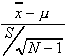, d.f. = N-1

Where:= mean of the sample= population mean

S = sample standard deviation

N = total number in the sample

d.f. = degrees of freedom

Assumption: Population mean known.

Example: Using the same data as above, except the sample size is now only 17, is the difference still significant?

t=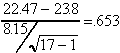, d.f. = 17-1=16

Look at the significant values for t-test chart for the one-tailed test..

-------P=.05 ---------P=.01

df

16----- 1.76 ----------2.583

P>.05, this average falls within the range that 95% of the means fall, the null hypothesis is NOT rejected. The difference in means is not significant and cannot be generalized to the population of students in 2:00 classes.

ONE SAMPLE T-TEST OF PROPORTIONS: Univariate inference for nominal data.

In many cases, analyst do not have interval/ratio level data. The following is an inferential test based on the same logic as the t-test of means, but using sample proportions and a known population proportion.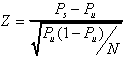where: Ps = Sample proportion

Pu = Population proportion

N = Sample total

Assumptions: N>100

Population proportion known.

Example: In Community A, 10.9% of the people know someone with AIDS. Taking a random sample of 104 people at the senior citizen center, only 5% of the sample knows someone with AIDS.

Q: Is this difference significant?

A:Look on the Z-chart, P<.05, therefore, the null hypothesis that there is no relationship between being a senior citizen and knowing someone with AIDS is rejected. Our conclusion is that fewer senior citizens people in Community A know people with AIDS than the rest of the population.

TWO-SAMPLE T-TESTS

TWO-SAMPLE T-TEST OF MEANS: Bivariate inferential for interval/ratio data

In this case, the population descriptive statistics are not necessary.

A. For Large Samples: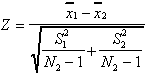Where: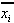= the mean of each sample.

Si = the standard deviation of each sample

Ni = sample total of each sample.

Assumptions: N > 100

Independent samples (this can be difficult to prove, assume independence for this class)

Example: In random samples of 143 female and 112 male SDSU students, the average hourly wage for female students is \$6.73 with a standard deviation of \$2.58. For the male students, it is \$7.72 with a standard deviation of \$4.79.

Q: Is this difference in hourly wages between male and female students generalizeable to the whole population of SDSU?

A: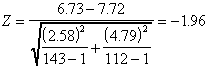Yes, it is generalize. p = .05.

There is a 5% chance of being wrong if we state that gender is associated with hourly wages of SDSU students.

B. For Small Samples: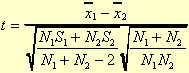d.f = N1 + N2 - 2.

Where:= the mean of each sample.

Si = the standard deviation of each sample

Ni = sample total of each sample.

d.f. = degrees of freedom.

Assumptions: Independent samples (this can be difficult to prove, assume independence for this class)

Similar Population variances (Similar variance can be proven by Bartlet's test, which is beyond the scope of this class, so assume similar variance as well)

Example: This time find out if there is a relationship between gender and hours spent working in the labor force within SDSU's student population. The sample average of 23 male students is 25 hours with a standard deviation of 9.27 hours. The sample average of 30 female students is 22.71 hours with a standard deviation of 8.15 hours.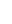A: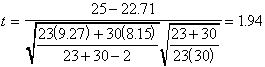d.f = 23 + 30 - 2 = 51Look in the back of the book for the critical values of the t-test with the a d.f. of 30.

d.f.----P=.05 --------P=.01

30 ------2.042-------- 2.75 for two-tailed

30 ------1.697 --------2.457 for one-tailed

Since the hypothesis did not indicate a direction (i.e. stating being a female negatively relates to hours worked in the labor force as compared to being a male), use the two-tailed critical values and fail to reject the null hypothesis that there is no relationship. Therefore, do not generalize. If the hypothesis had indicated a direction, using the one-tailed test would have given a probability value of less than 5% and the above difference in means could be generalized to the whole SDSU student population.

TWO-SAMPLE T-TEST OF PROPORTIONS: Bivariate inferential statistic for nominal data.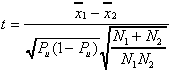where: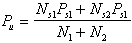= the mean of each sample.

Pi = the proportion of each sample

Ni = sample total of each sample.

Assumptions: N > 100

Example: 17.4% of 115 male and 27.3% of 132 females SDSU students randomly surveyed answered "yes" to the question "Do you know anyone who has contracted the HIV virus?"

Q: Can you generalize the difference to the whole SDSU student population?

A: First, estimate the population proportion.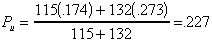Then calculate the standardized score.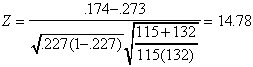P < .01, There is less than a one percent chance that there is no relationship between gender and knowing someone who has contracted the HIV virus among the SDSU student population. So yes, this sample's gender difference can be generalized to the whole population.